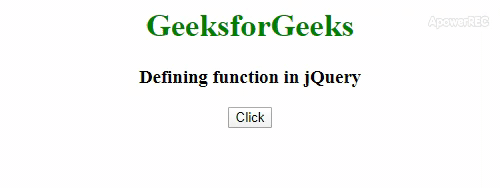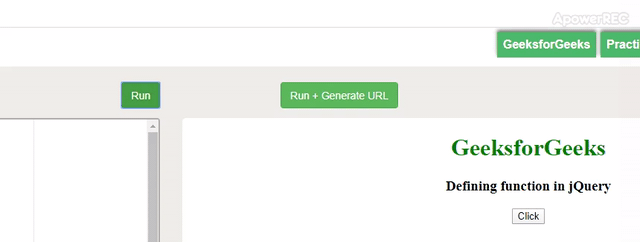# How to define jQuery function ?

Defining the function in jQuery is different from JavaScript, the syntax is totally different. A function is a set of statements that takes input, do some specific computation and produce output. Basically, a function is a set of statements that performs some specific task or does some computation and then return the result to the user.
The idea is to put some commonly or repeatedly done tasks together and make a function so that instead of writing the same code again and again for different inputs, we can call that function.

Syntax:

`\$.fn.myFunction = function(){}`

Below examples illustrate the function definition in jQuery:

Example 1:

 `  ` `<``html``>  ` ` `  `<``head``>  ` `    ``<``title``> ` `        ``How to Define jQuery function ? ` `    ``  ` `     `  `    ``<``script` `src``= ` `"https://code.jquery.com/jquery-1.12.4.min.js"``> ` `    `` ` `     `  `    ``<``script``> ` `        ``\$(document).ready(function() { ` `            ``\$.fn.myFunction = function() {  ` `                ``document.getElementById("geeks").innerHTML ` `                    ``= "JQuery function is defined!"; ` `            ``} ` `             `  `            ``\$(".gfg").click(function(){ ` `                ``\$.fn.myFunction(); ` `            ``}); ` `        ``}); ` `    `` ` `  ` ` `  `<``body` `style``=``"text-align:center"``>  ` ` `  `    ``<``h1` `style``=``"color:green;"``>  ` `        ``GeeksforGeeks  ` `    ``  ` `     `  `    ``<``h3``> ` `        ``Defining function in jQuery ` `    ``  ` `     `  `    ``<``p` `id``=``"geeks"``> ` `     `  `    ``<``button` `type``=``"button"` `class``=``"gfg"``> ` `        ``Click ` `    `` ` `  ` ` `  `         `

Output:Example 2:

 `  ` `<``html``>  ` ` `  `<``head``>  ` `    ``<``title``> ` `        ``How to Define jQuery function ? ` `    ``  ` `     `  `    ``<``script` `src``= ` `"https://code.jquery.com/jquery-1.12.4.min.js"``> ` `    `` ` `     `  `    ``<``script``> ` `        ``\$(document).ready(function() { ` `            ``\$.fn.myFunction = function() {  ` `                ``alert('JQuery function is defined!');  ` `            ``} ` `            ``\$(".gfg").click(function(){ ` `                ``\$.fn.myFunction(); ` `            ``}); ` `        ``}); ` `    `` ` `  ` ` `  `<``body` `style``=``"text-align:center;"``>  ` `     `  `    ``<``h1` `style``=``"color:green;"``>  ` `        ``GeeksforGeeks  ` `    ``  ` `     `  `    ``<``h3``> ` `        ``Defining function in jQuery ` `    ``  ` `     `  `    ``<``button` `type``=``"button"` `class``=``"gfg"``> ` `        ``Click ` `    `` ` `  ` ` `  `        `

Output:My Personal Notes arrow_drop_upSmall things always make you to think big

If you like GeeksforGeeks and would like to contribute, you can also write an article using contribute.geeksforgeeks.org or mail your article to contribute@geeksforgeeks.org. See your article appearing on the GeeksforGeeks main page and help other Geeks.

Please Improve this article if you find anything incorrect by clicking on the "Improve Article" button below.